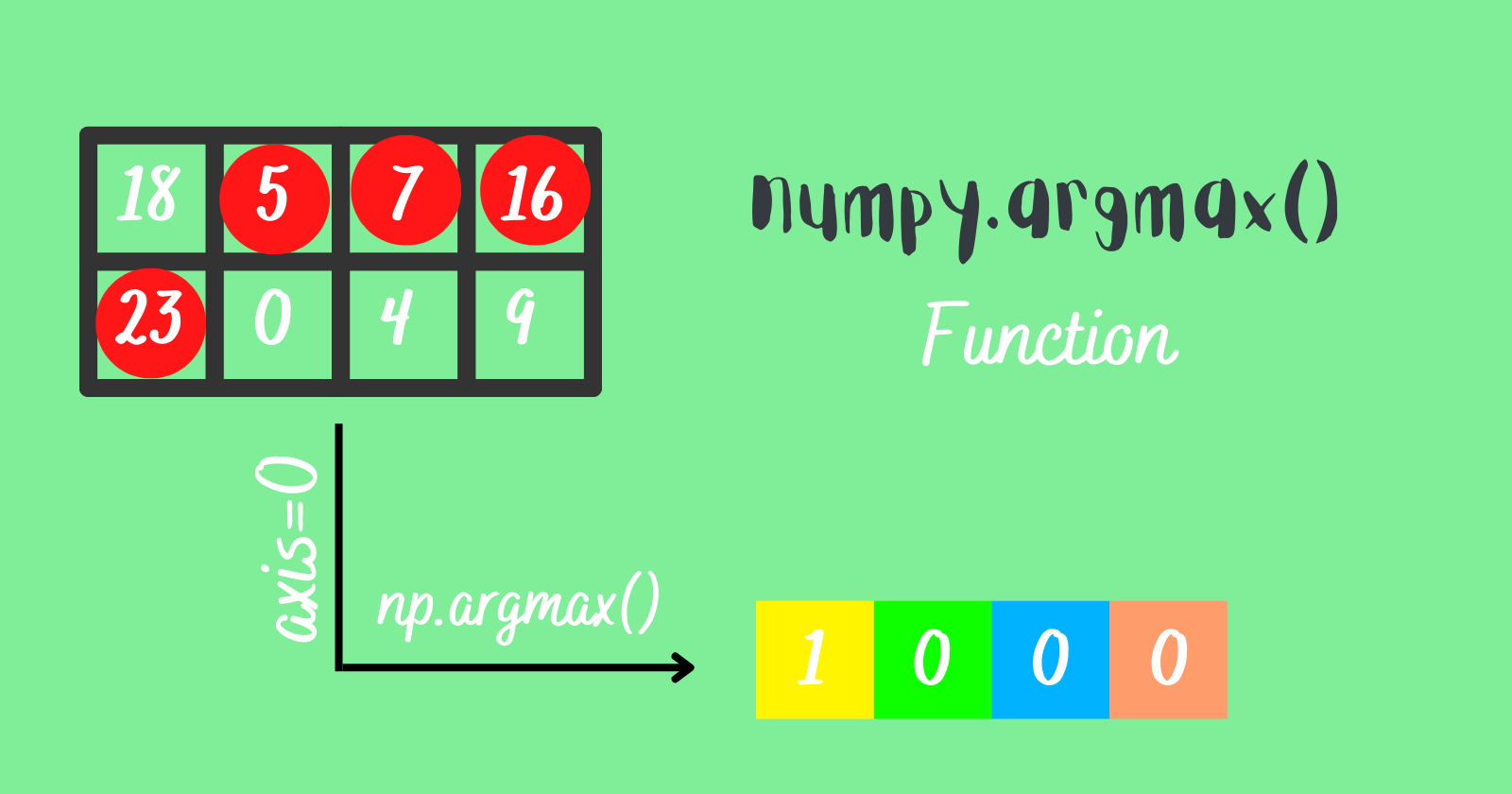## Understanding numpy.argmax() Function In Python

NumPy is most often used to handle or work with arrays (multidimensional, masked) and matrices. It has a collection of functions and methods to operate on arrays like statistical operations, mathematical and logical operations, shape manipulation, linear algebra, and much more.

# Argmax function

numpy.argmax() is one of the functions provided by NumPy that is used to return the indices of the maximum element along an axis from the specified array.

### Syntax

numpy.argmax(a, axis=None, out=None)

Parameters:

• `a` – The input array we will work on
• `axis` – It is optional. We can specify an axis like 1 or 0 to find the maximum value index horizontally or vertically.
• `out` – By default, it is None. It provides a feature to insert the output to the out array, but the array should be of appropriate shape and dtype.

Return value

The array of integers is returned with the indices of max values from the array `a` with the same shape as `a.shape` with the dimension along the axis removed.

### Finding the index of the max element

Let’s see the basic example to find the index of the max element in the array.

Working with a 1D array without specifying the axis

Output

Working with a 2D array without specifying the axis

When we work with 2D arrays in numpy and try to find the index of the max element without specifying the axis, the array we are working on has the element index the same as the 1D array or flattened array.

Output

### Finding the index of the max element along the axis

Things will change when we specify the axis and try to find the index of the max element along it.

When the axis is 0

When we specify the `axis=0`, then the argmax function will find the index of the max element vertically in the multidimensional array that the user specified. Let’s understand it by an illustration below.

In the above illustration, argmax() function returned the max element index from the 1st column which is 1 and then returned the max element index from the 2nd column which is again 1, and the same does for the 3rd and the 4th column.

Output

When the axis is 1

When we specify the `axis=1`, the argmax function will find the index of the max element horizontally in the multidimensional array that the user specified. Let’s understand it by an illustration below.

In the above illustration, argmax() function returned the max element index from the 1st row which is 2 and then returned the max element index from the 2nd row which is 1, and the same does for the 3rd and the 4th row.

Code example

Output

### Multiple occurrences of the highest value

Sometimes, we can come across multidimensional arrays with multiple occurrences of the highest values along the particular axis, then what will happen?

The argmax() function will return the index of the highest value that occurs first in a particular axis.

Illustration showing multiple occurrences of the highest values along axis 0.

Illustration showing multiple occurrences of the highest values along axis 1.

Code example

Output

Explanation

In the above code, when we try to find the indices of the max elements along the axis 0, we got an array with values `[2 0 1 1 2]`, if we look at the 2nd column, 14 is the highest value at the 0th and the 1st index, we got `0` because the value 14 at the 0th index occurred first when finding the highest value.

The same goes for the array we obtained in the second output when we provided the axis 1, in the 3rd row, 91 is the highest value at the 0th and the 4th index, the value 91 at the 0th index occurred first when finding the highest value hence we got output `0`.

### Using the out parameter

The `out` parameter in numpy.argmax() function is optional and by default it is None.

The `out` parameter stores the output array(containing indices of the max elements in a particular axis) in a numpy array. The array specified in the out parameter should be of shape and dtype, the same as the input array.

Code Example

Output

Explanation

We created an array filled with zeroes named `out_array`, and we defined the shape and dtype same as the input array and then used the `numpy.argmax()` function to get the indices of the max elements along the axis 1 and 0 and stored them in the `out_array` that we defined earlier.

The `numpy.zeros()` has by default dtype `float` that’s why we defined the dtype in the above code because our input array has the `dtype=int`.

If we didn’t specify the dtype in the above code, it would throw an error.

Output

# Conclusion

That was the insight of the argmax() function in NumPy. Let’s review what we’ve learned:

• `numpy.argmax()` function returns the index of the highest value in an array. If the maximum value occurs more than once in an array(multidimensional or flattened array), then the argmax() function will return the index of the highest value which occurred first.
• We can specify the axis parameter when working with a multidimensional array to get the result along a particular axis. If we specify axis=0, then we’ll get the index of the highest values vertically in the multidimensional array, and for axis=1, we’ll get the result horizontally in the multidimensional array.
• We can store the output in another array specified in the out parameter; however, the array should be compatible with the input array.

That’s all for now

Keep Coding✌✌